College Physics : Conservation of Momentum and Energy

Example Questions

Example Question #1 : Work And Energy

A toy car is set up on a frictionless track containing a downward sloping ramp and a vertically oriented loop. Assume the ramp is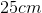tall. The car starts at the top of the ramp at rest.

What additional piece of information is necessary to calculate the maximum height of the loop if the car is to complete the loop and continue out the other side?

The mass of the car

The exact shape of the loop

The value of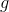None

The distance between the end of the ramp and entrance to the loop

None

Explanation:

This is an example of conservation of energy. The car starts at the top of the ramp, at height. It has no velocity at this time since it is starting from a rest. Therefore its total energy is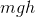whereis the mass of the car andis the value of gravitational acceleration.

At the bottom of the loop, all of the potential energy will have been converted into kinetic energy.

As the car traverses the loop and rises above the ground, kinetic energy will be converted back into potential energy. The shape of the loop does not matter in this case -- only the vertical distance between the ground and the car.

In the tallest possible loop, all kinetic energy at the bottom is converted to potential energy at the top. This is the maximum height the car can reach -- there is no additional energy left to continue climbing a taller loop. Therefore, the potential energy at the top of the tallest loop we can build is equal to the kinetic energy at the bottom of the loop. But we have already noted that the kinetic energy at the bottom of the loop is equal to the potential energy at the top of the ramp.

Therefore, we set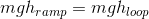We see thatandcancel, and we are left with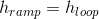. In other words, the tallest loop you can build is equal to the height of whatever ramp you select. In this example, the tallest loop we can build is. We do not need to know the specific values ofor.

Example Question #1 : Conservation Of Momentum And Energy

An elevator is designed to hold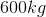of cargo. The designers want the elevator to be able to go from the ground floor to the top of a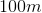tall building in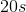. What is the minimum amount of power that must be delivered to the motor at the top of the shaft? Assume no friction and that the elevator itself has a negligible weight.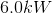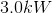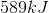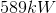Explanation:

Power is the rate of energy transfer. To raise aobject, a total ofor (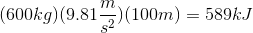is required. To find the power in Watts (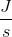), we divide the total energy required by the time over which the energy must be transferred: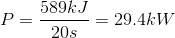Example Question #1 : Mechanics

How far can a person jump while running at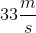and a vertical velocity of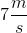?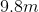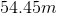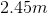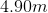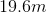Explanation:

We know that: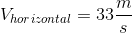and we are looking for the maximum height (vertical displacement) this person can obtain, so we aren't concerned with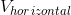.

We can apply the conservation of energy: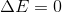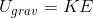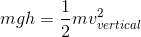Masses cancel, so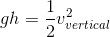Solve for: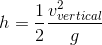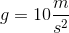(rounded to simplify our calculations)

so let's plug in what we know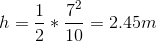. This is our final answer.

Example Question #2 : Conservation Of Momentum And Energy

If a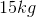object has a kinetic energy of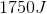right after it is launched in the air, and it has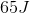KE at its max height, what is its max height?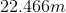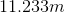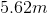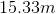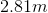Explanation:

Let's first write down the information we are given: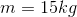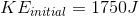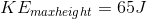In order to solve this problem we must apply the conservation of energy, which statessince no friction.

This means that as the project reaches its max height energy is converted from Kinetic Energy (energy of motion) to potential gravitational energy (based off of height).

We can subtract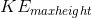from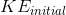to get the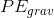at its max height=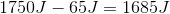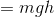so we can solve for the height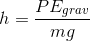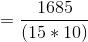wheretherefore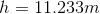Example Question #21 : College Physics

If an object has a kinetic energy of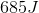right after it is launched in the air, and it has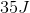KE at its max height of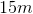, what is the object's mass?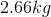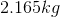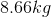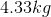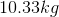Explanation:

Let's first write down the information we are given: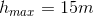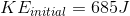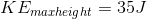In order to solve this problem we must apply the conservation of energy, which statessince no friction.

This means that as the project reaches its max height energy is converted from Kinetic energy (energy of motion) to potential gravitational energy (based off of height).

We can subtractfromto get theat its max height=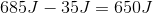so we can solve for the mass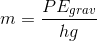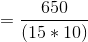wheretherefore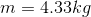All College Physics Resources